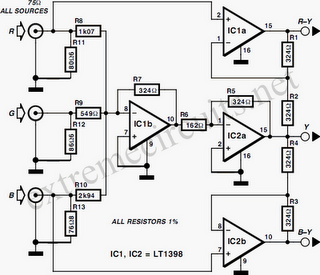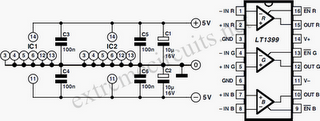# Learning Electronics

Learn to build electronic circuits

# RGB To Color Difference Converter

The circuit diagram shows two LT1398’s from Linear Technology used to create buffered color-difference signals from RGB (red-green-blue) inputs. In this application, the R input arrives via 75Ω coax. It is routed to the non-inverting input of amplifier IC1a and to 1.07-kΩ resistor, R8. There is also an 80.6-Ω termination resistor R11, which yields a 75-Ω input impedance at the R input when considered in parallel with R8. R8 connects to the inverting input of a second LT1398 amplifier (IC1b), which also sums the weighted G and B inputs to create a –0.5Y output.Yet another LT1398 amplifier, IC2a, then takes the –0.5Y output and amplifies it by a gain of –2, resulting in the +Y output. Amplifier IC1a is configured for a non-inverting gain of 2 with the bottom of the gain resistor R2 tied to the Y output. The output IC1a thus results in the color-difference output R–Y. The B input is similar to the R input. Here, R13 when considered in parallel with R10 yields a 75-Ω input impedance. R10 also connects to the inverting input of ampliﬁer IC1b, adding the B contribution to the Y signal as discussed above.Amplifier IC2b is conﬁgured to supply a non-inverting gain of 2 with the bottom of the gain resistor R4 tied to the Y output. The output of IC2b thus results in the color-difference output B–Y. The G input also arrives via 75-Ω coax and adds its contribution to the Y signal via resistor R9, which is tied to the inverting input of amplifier IC1b. Here, R12 and R9 provide the 75Ω termination impedance. Using superposition, it is straightforward to determine the output of IC1b. Although inverted, it sums the R, G and B signals to the standard proportions of 0.3R, 0.59G and 0.11B that are used to create the Y signal. Amplifier IC2a then inverts and amplifies the signal by 2, resulting in the Y output. The converter draws a current of about 30mA from a symmetrical 5-volt supply.